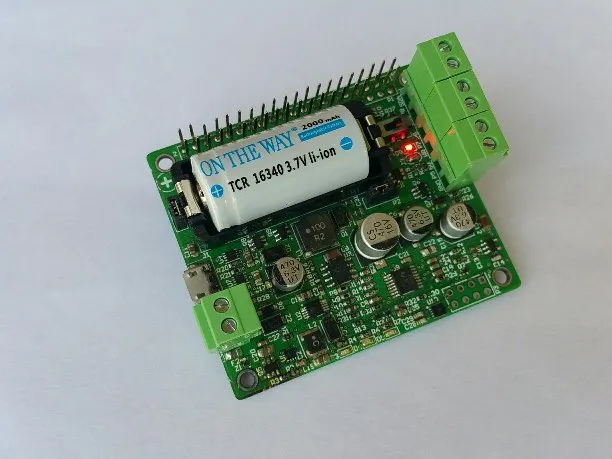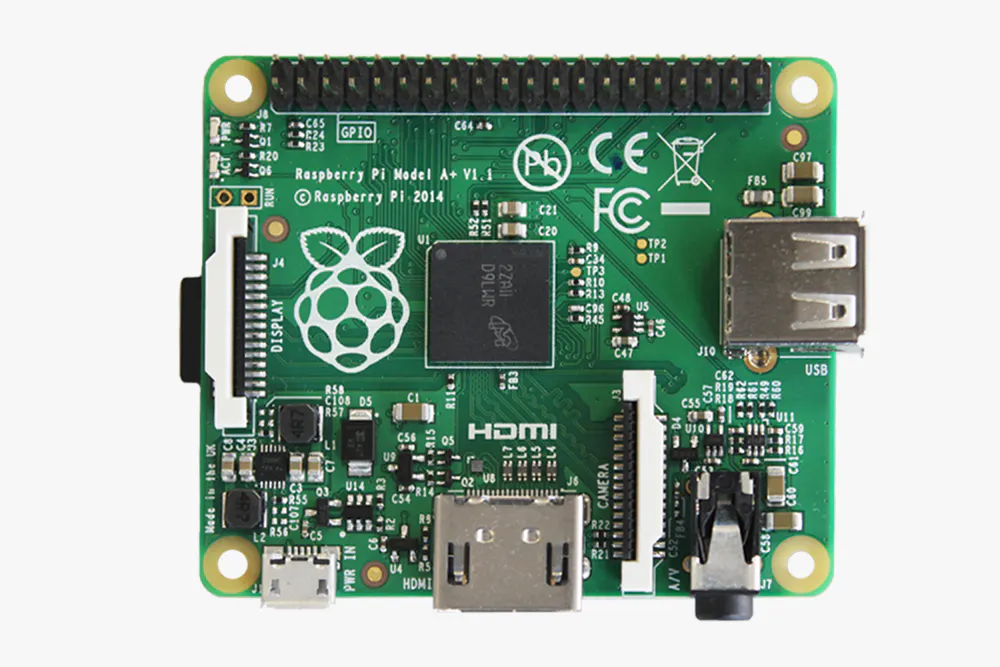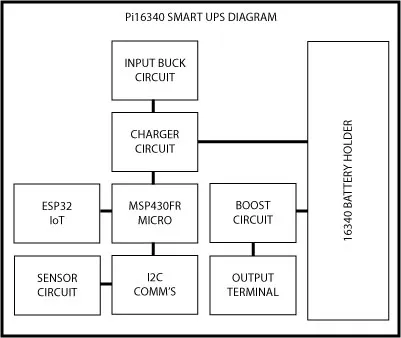Published

# Pi16340 Smart UPS for Raspberry PI 3A+

A compact, feature-rich smart UPS for Raspberry Pi boards.

IntermediateProtip1 hour1,576## Things used in this project

### Hardware componentsRaspberry Pi 1 Model A+
×1

### Hand tools and fabrication machinesMultitool, Screwdriver Small flat head screwdriver for terminal blocks.

## Schematics

### Pi16340 Block Diagram

Outline of the various sections that compose the control and power circuitry.## Code

### Monitoring Software

Python
This Python code runs on the Raspberry Pi to read status from the Pi16340 SMART UPS.
```#!/usr/bin/env python

import io
import fcntl
import struct
import time

I2C_SLAVE=0x0703

class i2c:

def __init__(self, device, bus):

self.fr = io.open("/dev/i2c-"+str(bus), "rb", buffering=0)
self.fw = io.open("/dev/i2c-"+str(bus), "wb", buffering=0)

fcntl.ioctl(self.fr, I2C_SLAVE, device)
fcntl.ioctl(self.fw, I2C_SLAVE, device)

def write(self, bytes):
self.fw.write(bytes)

def close(self):
self.fw.close()
self.fr.close()

def get_int(self, var_slice):

ms = memoryview(var_slice)
var_list = map(ord,ms)
#print("bat_list: ", bat_list)
var_int = var_list*256 + var_list

return var_int

def get_single_int(self, var_slice):

ms = memoryview(var_slice)
var_list = map(ord,ms)
#print("bat_list: ", bat_list)
var_int = var_list

return var_int

#<<----------------------------------------------------------------------------------------
#comment out this section below if you would like to import the class into your own program
#---------------------------------------------------------------------------------------->>
testpoll = i2c(0x48,1)
samples = 5
bat_avg = 0
hum_avg = 0
temp_avg = 0
sample_delay = 0.010

for i in range(samples):
print("result: ", result)

bat = result[:2]
hum = result[2:4]
temp = result[4:6]
mode = result[6:7]
reboot = result[7:8]
pwrlvl = result[8:9]
temp2 = result[-1:]

#print("bat: ", bat)
#print("hum: ", hum)
#print("temp: ", temp)

for j in range(6):
if j == 0:
res = testpoll.get_int(bat)
bat_avg = bat_avg + res

elif j == 1:
res1 = testpoll.get_int(hum)
hum_avg  = hum_avg + res1

elif j == 2:
res2 = testpoll.get_int(temp)
temp_avg  = temp_avg + res2

elif j == 3:
mode_res = testpoll.get_single_int(mode)

elif j == 4:
reboot_res = testpoll.get_single_int(reboot)

elif j == 5:
pwrlvl_res = testpoll.get_single_int(pwrlvl)
else:
no_op = ""

result = '0'
res = 0
res1 = 0
res2 = 0
time.sleep(sample_delay)

#m = memoryview(bat)
#bat_list = map(ord,m)
#print("bat_list: ", bat_list)

#Battery Level calculation---------------
#bat_int = bat_list*256 + bat_list
#print("bat_int: ", bat_int)

bat_avg_int = bat_avg / samples
battery = (float(bat_avg_int) * 0.00322) / 0.7674
print "Battery: ", battery ,"V"
#----------------------------------------

#m1 = memoryview(hum)
#hum_list = map(ord,m1)
#print("hum_list: ", hum_list)

#Humidity calculation--------------------
#hum_int = hum_list*256 + hum_list
#print("hum_int: ", hum_int)

humidity = 0.0
hum_avg_int = hum_avg / samples
humidity = float(-12.5) + (float(125) * (float(hum_avg_int) / float(1024)))
humidity  = round(humidity,2)
print "Humidity: ", humidity ,"%"
#----------------------------------------

#m2 = memoryview(temp)
#temp_list = map(ord,m2)
#print("temp_list: ", temp_list)

#Temperature calculation - Deg C-----------
#temp_int = temp_list*256 + temp_list
#print("temp_int: ", temp_int)

temperature = 0.0
temp_avg_int = temp_avg / samples
temperature = float(-66.875) + (float(218.75) * (float(temp_avg_int) / float(1024)))
temperature = round(temperature,2)
print "Temperature: ", temperature ,"C"
#-----------------------------------------

#Temperature calculation - Deg F-----------
#temp_int = temp_list*256 + temp_list
#print("temp_int: ", temp_int)

temperatureF = 0.0
temp_avg_int = temp_avg / samples
temperatureF = float(-88.375) + (float(393.75) * (float(temp_avg_int) / float(1024)))
temperatureF = round(temperatureF,2)
print"Temperature: ", temperatureF ,"F"
#-----------------------------------------

#mode = mode.strip()
#reboot = reboot.strip()
#mode = mode.replace('\\', ' ')
#reboot = reboot.replace('\\', ' ')

if mode_res == 0:
print"Mode: Battery(", mode_res, ")"
else:
print"Mode: UPS(", mode_res, ")"

reboot_res = reboot_res >> 4
print"Reboot Status: ", reboot_res

pwrlvl_res = pwrlvl_res >> 5
print"Supply Power Level Status: ", pwrlvl_res

#dev.write("\x2D\x00") # POWER_CTL reset

testpoll.close()
```

## Credits

### Mark Chin

5 projects • 10 followers
Entrepreneur and Innovator of embedded systems, RFID and IoT.